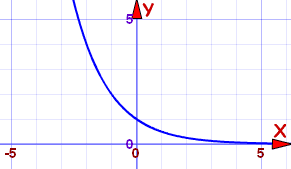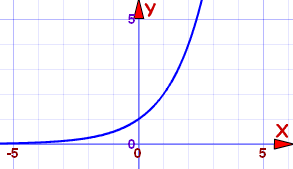# 指数函数参考

f(x) = ax

a 是任何大于 0 的数

### 函数的属性也和 "a" 的值有关系

• a=1 时，函数的图是穿过 y=1 的水平线
• 此外，有两个情形需要考虑：

a 在 0 与 1 之间a 在 0 与 1 之间

• x 的值越大，f(x) 就越趋向 0
• x 的值越小，f(x) 就越趋向无穷大
• 它是个严格递减函数（因此是 "单射" 函数）
• x轴（y=0）是函数的水平渐近线

a 大于 1a 大于 1：

• x 的值越大，f(x) 就越趋向无穷大
• x 的值越小，f(x) 就越趋向 0
• 它是个严格递增函数（因此是 "单射" 函数）
• x轴（y=0）是函数的水平渐近线

### 一般来说：

• 指数函数的值永远大于 0，并且永不穿过 x轴
• 函数的曲线与 y轴在 y=1 相交 …… 即是线穿过 (0,1)
• x=1f(x)=a …… 即是线穿过 (1,a)
• 是个单射（一对一）函数

## 反函数

ax 是 loga(x)对数函数）的反函数

## 自然指数函数

f(x) = exf(x) = ex 的图